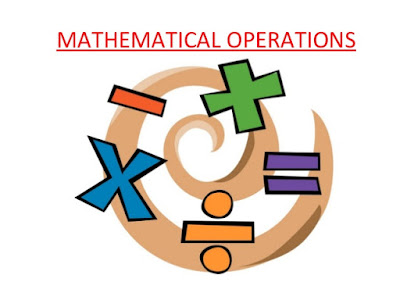# Mathematical Operations :-

Mathematical Operations Practice Questions section is below. Here, the four fundamental operations — addition, subtraction, multiplication and division and also statements such as less than, ‘greater than’, ‘equal to’, ‘not equal to1, etc. are represented by symbols, different from the usual ones. The questions involving these operations are set using artificial symbols. The candidate has to substitute the real signs and solve the questions accordingly, to get the answer.

In this type of questions, usual mathematical symbols (+-,÷, x,<,> etc.) are represented by symbols, different from the usual ones. To solve this type of questions, substitute the real signs in the given expression and then solve the expression according to the rule BODMAS.Mathematical Operations

Q1: If the given interchanges namely : signs + and + and numbers 2 and 4 are made in signs and numbers, which one of the following four equations would be correct?
(a) 2 + 4 + 3 = 3             (b) 4 + 2 + 6 = 1.5
(c) 4 + 2 + 3 = 4           (d) 2 + 4 + 6 = 8

Q2: Which one of the four interchanges in signs and numbers would make the given equation correct? 3 + 5 – 2 = 4
(a) + and 2 and 3          (b) + and 2 and 5
(c) + and 3 and 5           (d) None of these

Directions (Questions 3 to 6): In each of the following questions, which one of the four interchanges in signs and numbers would make the given equation correct

Q3. 6 x 4 + 2=16
(a) + and x, 2 and 4         (b) + and x, 2 and 6
(c) + and x, 4 and 6        (d) None of these

Q4. (3+ 4)+ 2 = 2
(a) + and +, 2 and 3          (b) + and +, 2 and 4
(c) + and +, 3 and 4       (d) No interchange, 3 and 4

Q5. 4 x 6 – 2 – 14
(a) x to +, 2 and 4            (b) – to +, 2 and 6
(c) – to +, 2 and 6             (d) x to +, 4 and 6

Q6. (6 +2) x 3 – 0
(a) + and x, 2 and 3         (b) x to 2 and 6
(c) + and x, 2 and 6                (d) x to 2 and 3

Find Your Answers Here

Q1: (d), Q2: (c), Q3: (c), Q4: (a), Q5: (c), Q6: (d)